# [How-to] Share content between several R6 instancesThe object oriented system is a nice and powerful way to program and to build softwares and data in R. Yet, it can be a little bit daunting at first, especially if you’ve alway been coding in R, and with functions. In this blogpost, we’ll have a look at {R6}, one of the most downloaded package from the CRAN, which is one of the backbone of a lot of package backends, and specifically answer the question of data sharing across class instances.

## What is {R6}?

In a few words, {R6} is a modern and flexible Object Oriented (“OO”) framework for R.

So yes, your next question might be: what does “object oriented” mean? With OO, you’re creating objects, and inside these objects are data and methods (i.e, function). Running these methods can change the content of the data contained inside the object.

As in any OO system, {R6} has a system of classes and instances of these classes — which is the subject of this post: how can you share content between every instances of a class?

## When should we need {R6}?

One main use case is when you need to build an object, and you want to enclose inside this object information, but also perform a series of actions on these information. You could do this by defining functions and objects and use all these objects together. But the idea here is to have something that is enclosed inside the same object, and all the objects from the same class starts with the same content and methods.

R6 can be used to deal with database connection, for example. Inside the R6 object, you could find all the information about the connection, and also methods specifically related to interacting with the database. You can also built documents, which are constructed inside the object.

Here are some examples of packages using an R6-oriented API:

## Playing with {R6}

We will create an R6 class to generate HTML and CSS code, in order to have a webpage.

Disclaimer: of course, this R6 class is (really) limited for webpage generation, the idea here is to provide an example, not to write a prod-ready website generator 😉

``````library(R6)
library(glue)
library(purrr)
library(magrittr)
library(htmltools)

WebPage <- R6Class("WebPage",
public = list(
name = character(0),
body = "<body>",
style = '<style type="text/css">',
content <- imap_chr(content, ~ glue("{.y} : {.x};")) %>%
unname() %>%
paste(collapse = " ")
glued <- glue("%identifier% { %content% }",
.open = "%", .close = "%")
self\$style <- c(self\$style, glued)
},
initialize = function(name){
self\$name <- name
},
glued <- glue("<{tag}>{content}</{tag}>")
self\$body <- c(self\$body, glued)
},
save = function(path){
glue("{file.path(path, self\$name)}.html"))
},
view = function(){
},
print = function(){
cat(private\$concat(self\$head, self\$style, self\$body), sep = "\n")
}
),
private = list(
}
)
)

a <- WebPage\$new("index")

a\$add_style("body", list("font-family" = "Helvetica", "color" = "#24292e"))
a\$add_style("h2", list("font-size" = "3 em", "color" = "#911414",
"text-align" = "center"))
a\$add_style("h3", list("font-size" = "1.5 em", "color" = "#2E2E8F",
"text-align" = "center"))

a``````
``````<!DOCTYPE html>
<html>
<style type="text/css">
body { font-family : Helvetica; color : #24292e; }
h2 { font-size : 3 em; color : #911414; text-align : center; }
h3 { font-size : 1.5 em; color : #2E2E8F; text-align : center; }
</style>
<body>
<h2>Hey there!</h2>
<p>We are glad to have you here.</p>
</body>
</html>``````
``````a\$view()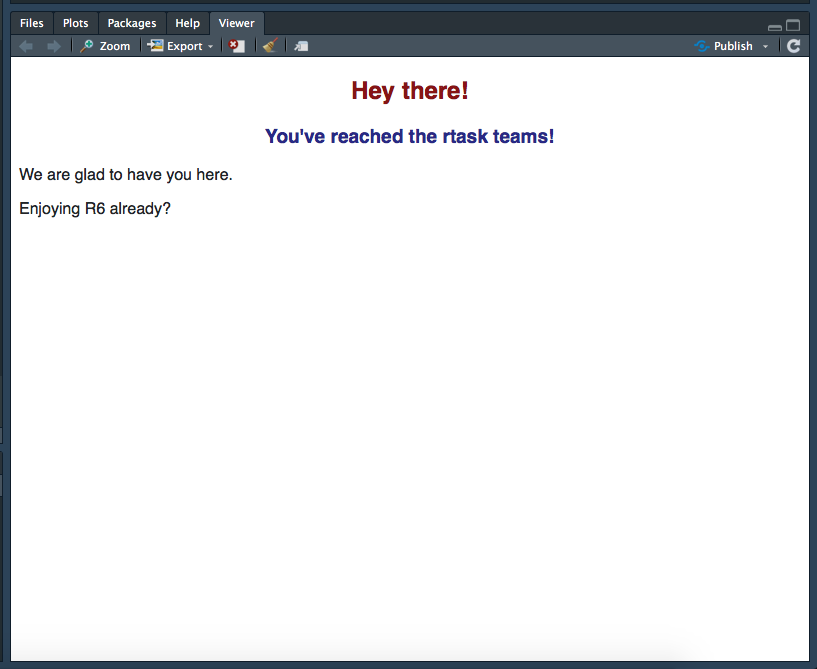a\$save(".")``````

Ok, so let’s cut everything we did into pieces:

``WebPage <- R6Class("WebPage", ``

Here, we are giving a name to our class. By convention, it should be CamelCase.

``````                   public = list(
name = character(0),
body = "<body>",
style = '<style type="text/css">',``````

With this lines of code, I’m creating a `public` list, which is basically the list of elements that are accessible to the user once the object is created.

``````                     initialize = function(name){
self\$name <- name
},``````

`initialize` is the function that is called when you do `class\$new(arg = )`. Here, we chose to give `self\$name` a name, that will be used later on with the `save` method.

``````                     add_style = function(identifier, content){
content <- imap_chr(content, ~ glue("{.y} : {.x};")) %>%
unname() %>%
paste(collapse = " ")
glued <- glue("%identifier% { %content% }",
.open = "%", .close = "%")
self\$style <- c(self\$style, glued)
},``````

This function is used to add a css element to style. For example, when I do :

``````a\$add_style("h3", list("font-size" = "1.5 em",
"color" = "#2E2E8F",
"text-align" = "center"))``````

I want the output to be:

``````h3 {font-size : 1.5 em;
color = #2E2E8F;
text-align = center;}``````

So this is what I do here: on the named list given as a parameter, I `imap` a custom function that will create, for each elements of the list, a `id : param;` element.

Then, this is put inside a `style { content }`, and as I need to use `{` as a real character, I’m using `%` to provide an opening and closing character (as `{glue}` uses `{` to mark the element to unquote by default, and this can be overridden).

``````                     add_tag = function(tag, content){
glued <- glue("<{tag}>{content}</{tag}>")
self\$body <- c(self\$body, glued)
},``````

Easy one here, just creating html tag content 😉

``````                      save = function(path){
self\$style,
self\$body),
glue("{file.path(path, self\$name)}.html")
)
},``````

This creates a function to save the html page, so basically just a write, with a path.

``````                     view = function(){
self\$style,
self\$body)))
},``````

This one is used to view the content of the page inside your default browser. I made the `concat` a private method (it concatenates the elements), `HTML` turns characters into HTML, and `html_print` shows the content.

``````                     print = function(){
cat(private\$concat(self\$head, self\$style, self\$body), sep = "\n")
}
), ``````

There is a default printing method for R6 objects, but it can be overridden. This is what I do here: specify what happen when the defined object is printed to the console.

``````                   private = list(
c( head, style, "</style>", body, "</body>","</html>")
}
)
)``````

And finally, our private list. I chose to put `concat` in here as I didn’t want nor need this function to be accessible outside the object, I could also have put it outside the class.

# Several pages, one CSS

It would be better if every instance of the class shared the same CSS, right?

So, here’s the thing: once an object is instantiated, its public objects are sealed: if A and B are instances of the C class, changing something in the public list of A won’t change it in B. And if you change something in C, that won’t “roll down” to the instances of the class. Which is a behavior you could expect: if I change the title of A, I don’t want the title of B to be changed.

But in our case, we need to be able to access the same data from multiple instances: in other word, I want all my instances of WebPages to access the very same CSS code. To do this, we’ll use R environments. In the private field of our R6 class definition, we’ll set up an environment in the `shared` field. Then, everytime I will create a new object of this class, the `shared` variable will point to the very same environment (and in fine, to the same CSS content).

Let’s do this:

``````WebSkeleton <- R6Class("WebSkeleton",
public = list(
name = character(0),
body = "<body>",
content <- imap_chr(content, ~ glue("{.y} : {.x};")) %>%
unname() %>%
paste(collapse = " ")
glued <- glue("%identifier% { %content% }",
.open = "%", .close = "%")
private\$shared\$style <- c( private\$shared\$style, glued)
},
glued <- glue("<{tag}>{content}</{tag}>")
self\$body <- c(self\$body, glued)
},
initialize = function(name){
self\$name <- name
},
save = function(path){
glue("{file.path(path, self\$name)}.html")
)
},
view = function(){
},
print = function(){
sep = "\n")
}
),
private = list(
},
shared = {
env <- new.env()
env\$style <- '<style type="text/css">'
env
}
)
)

# Create an index page
index <- WebSkeleton\$new("index")

"color" = "#24292e"))
index\$add_style("h2", list("font-size" = "3 em", "color" = "#911414",
"text-align" = "center"))
index\$add_style("h3", list("font-size" = "1.5 em", "color" = "#2E2E8F",
"text-align" = "center"))

index``````
``````<!DOCTYPE html>
<html>
<style type="text/css">
body { font-family : Helvetica; color : #24292e; }
h2 { font-size : 3 em; color : #911414; text-align : center; }
h3 { font-size : 1.5 em; color : #2E2E8F; text-align : center; }
</style>
<body>
<h2>Hey there!</h2>
<p>We are glad to have you here.</p>
</body>
</html>``````
``````index\$view()# Initiate a new page
team <- WebSkeleton\$new("team")
# Don't assign any style
team\$add_tag("h3", "Time to meet the team!")

team\$view()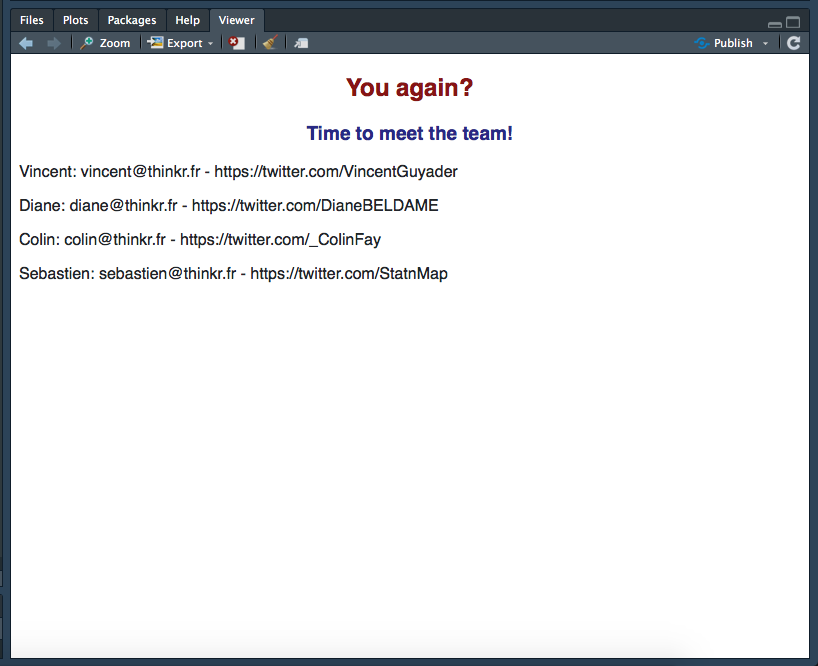``````

As you can see, I didn’t have to assign any style to get access to the CSS. And if I add another style to my team object:

``````team\$add_style("p", list("font-weight" = "bold"))
team\$view()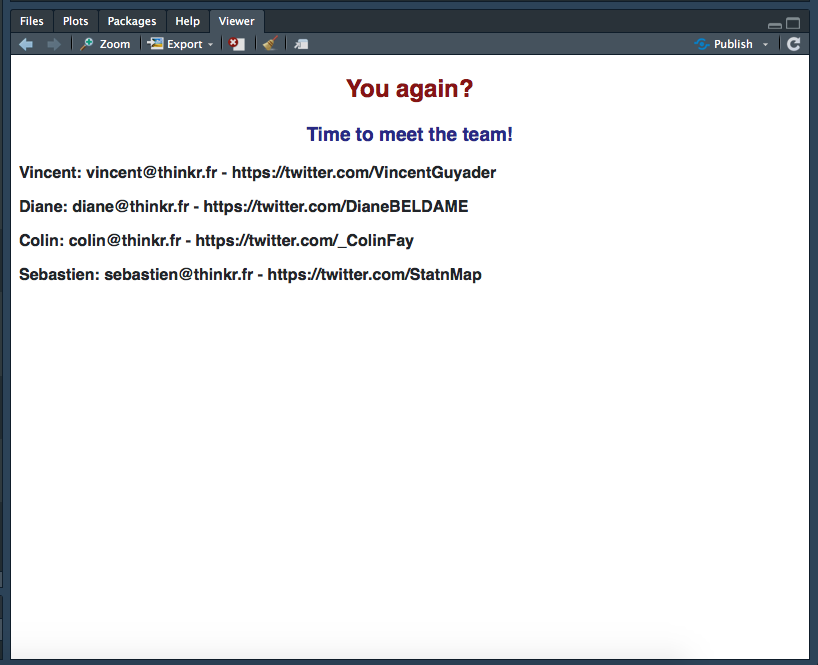``````

It will be shared to the `index` page.

``````index\$view()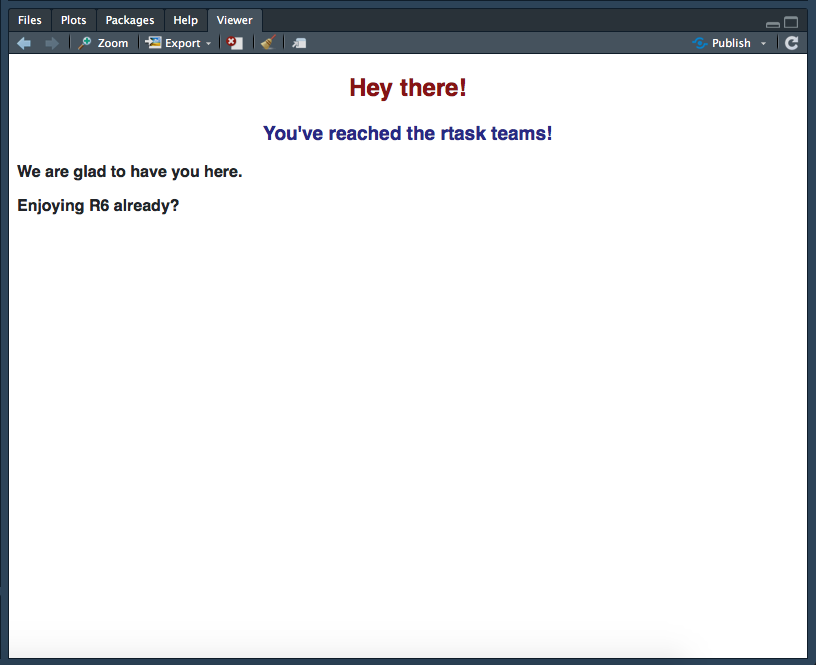``````

🎉

The CSS is now shared between all instances of the `"WebSkeleton"` class.

## How does this work?

So, here’s a quick demo to show you that public elements are not shared between class instances, but that environments are.

``````plop <- R6Class("plop",
public = list(a = 1,
change_a = function(val) { self\$a <- val },
print_b = function() { private\$shared\$b },
change_b = function(val) { private\$shared\$b <- val} ),
private = list( shared = { e <- new.env(); e\$b <- 2; e } )
)

plop_1 <- plop\$new()
plop_1\$a
 1

plop_2 <-  plop\$new()
plop_2\$change_a(12)
plop_2\$a
 12

plop_1\$a
 1

plop_1\$print_b()
 12
plop_2\$print_b()
 12

plop_1\$change_b(19)
plop_1\$print_b()
 19
plop_2\$print_b()
 19
``````

Here, once `plop_1<\code> is initialized, it contains the variable a with a specific value. If I initiate plop_2 and change_a<\code>, the only value that is changed is the one inside plop_2. But when it comes to b, as shared is an environment — and that environment is copied by reference, not by value —, we can share data across all instances.`

Conceptually speaking, each instance of the `a` variable points to its own space in memory, while all the `shared` elements point to the same spot, hence contain the same value.Colin Fay

Data scientist & R Hacker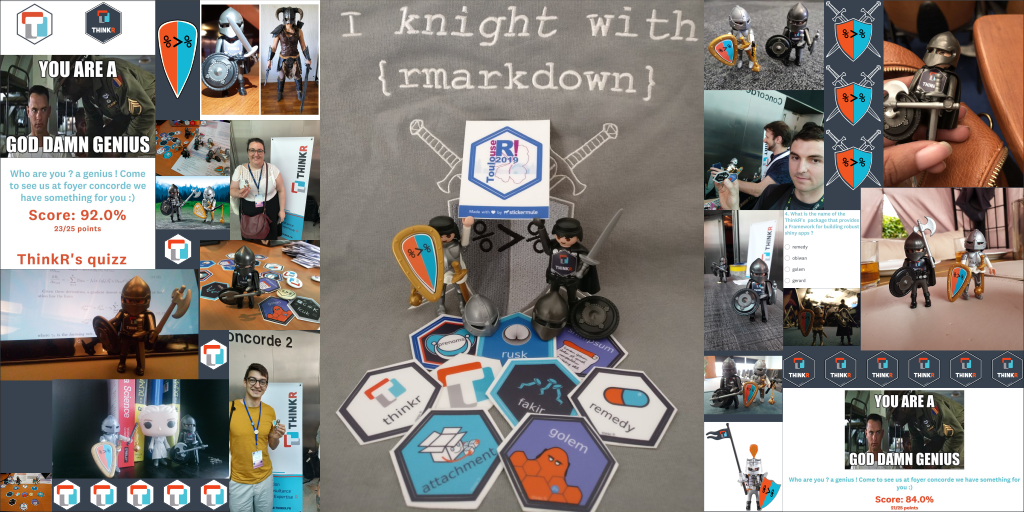#### useR!2019 Quizz: Test your knowledge of base R and ThinkR

2019-07-22 / Sébastien Rochette

13/04/2020

11/05/2020

11/03/2020

27/02/2020

05/02/2020